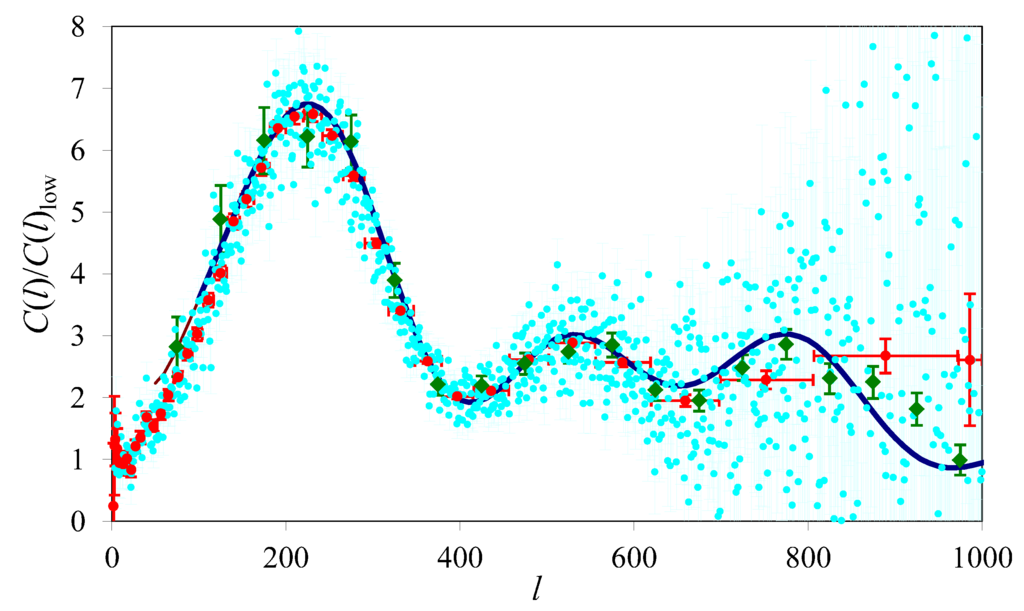Not for the first time, I am reading a paper that discusses the dark matter paradigm and its alternatives.

Except that it doesn’t. Discuss the alternatives, that is. It discusses the one alternative every schoolchild interested in the sciences knows about (and one that, incidentally, doesn’t really work) while ignoring the rest.

This one alternative is Mordehai Milgrom’s MOND, or MOdified Newtonian Dynamics, and its generalization, TeVeS (Tensor-Vector-Scalar theory) by the late Jacob Bekenstein.

Unfortunately, too many people think that MOND is the only game in town, or that even if it isn’t, it is somehow representative of its alternatives. But it is not.

In particular, I find it tremendously annoying when people confuse MOND with Moffat’s MOG (MOdified Gravity, also MOffat Gravity). Or when similarly, they confuse TeVeS with STVG (Scalar-tensor-Vector Gravity), which is the relativistic theory behind the MOG phenomenology.

So how do they differ?

MOND is a phenomenological postulate concerning a minimum acceleration. It modifies Newton’s second law: Instead of $$F = ma$$, we have $$F = m\mu(a/a_0)a$$, where $$\mu(x)$$ is a function that satisfies $$\mu(x)\to 1$$ for $$x\gg 1$$, and $$\mu(x)\to x$$ for $$x\ll 1$$. A good example would be $$\mu(x)=1/(1+1/x)$$. The magnitude of the MOND acceleration is $$a_0={\cal O}(10^{-10})~{\rm m}/{\rm s}$$.

The problem with MOND is that in this form, it violates even basic conservation laws. It is not a theory: it is just a phenomenological formula designed to explain the anomalous rotation curves of spiral galaxies.

MOND was made more respectable by Jacob Bekenstein, who constructed a relativistic field theory of gravity that approximately reproduces the MOND acceleration law in the non-relativistic limit. The theory incorporates a unit 4-vector field and a scalar field. It also has the characteristics of a bimetric theory, in that a “physical metric” is constructed from the true metric and the vector field, and this physical metric determines the behavior of ordinary matter.

In contrast, MOG is essentially a Yukawa theory of gravity in the weak field approximation, with two twists. The first twist is that in MOG, attractive gravity is stronger than Newton’s or Einstein’s; however, at a finite range, it is counteracted by a repulsive force, so the gravitational acceleration is in fact given by $$a = GM[1+\alpha-\alpha(1+\mu r)e^{-\mu r}]$$, where $$\alpha$$ determines the strength of attractive gravity ($$\alpha=0$$ means Newtonian gravity) and $$\mu$$ is the range of the vector force. (Typically, $$\alpha={\cal O}(1)$$, $$\mu^{-1}={\cal O}(10)~{\rm kpc}$$.) The second twist is that the strength of attractive gravity and the range of the repulsive force are both variable, i.e., dynamical (though possibly algebraically related) degrees of freedom. And unlike MOND, for which a relativistic theory was constructed after-the-fact, MOG is derived from a relativistic field theory. It, too, includes a vector field and one or two scalar fields, but the vector field is not a unit vector field, and there is no additional, “physical metric”.

In short, there is not even a superficial resemblance between the two theories. Moreover, unlike MOND, MOG has a reasonably good track record dealing with things other than galaxies: this includes globular clusters (for which MOND has to invoke the nebulous “external field effect”), cluster of galaxies (including the famous Bullet Cluster, seen by some as incontrovertible proof that dark matter exists) and cosmology (for which MOND requires something like 2 eV neutrinos to be able to fit the data.)MOG and the acoustic power spectrum. Calculated using $$\Omega_M=0.3$$, $$\Omega_b=0.035$$, $$H_0=71~{\rm km}/{\rm s}/{\rm Mpc}$$. Also shown are the raw Wilkinson Microwave Anisotropy Probe (WMAP) three-year data set (light blue), binned averages with horizontal and vertical error bars provided by the WMAP project (red) and data from the Boomerang experiment (green). From arXiv:1104.2957.

There are many issues with MOG, to be sure. Personally, I have never been satisfied with the way we treated the scalar field so far, and I’d really like to be able to derive a proper linearized version of the theory in which the scalar field, too, is accommodated as a first-class citizen. How MOG stands up to scrutiny in light of precision solar system data at the PPN level is also an open question.

But to see MOG completely ignored in the literature, and see MOND used essentially as a straw man supposedly representing all attempts at creating a modified gravity alternative to dark matter… that is very disheartening.